﻿ 二维地震波场的组合型紧致有限差分数值模拟
 地球物理学报2018, Vol. 61Issue (11): 4568-4583PDF

1. 油气资源与勘探技术教育部重点实验室(长江大学), 武汉 430100;
2. 长江大学地球物理与石油资源学院, 武汉 430100;
3. 胜利油田勘探开发研究院西部分院, 山东东营 257001

Numerical simulation of 2D seismic wave-field used combined compact difference scheme
WANG Yong1,2, SHI HaoGuo3, ZHOU ChengYao1,2, GUI ZhiXian1,2
1. Key Laboratory of Exploration Technologies for Oil and Gas Resources, Ministry of Education, Yangtze University, Wuhan 430100, China;
2. College of Geophysics and Petroleum Resources, Yangtze University, Wuhan 430100, China;
3. West Branch of Shengli Oilfield Exploration and Development Research Institute, Shandong Dongying 257001, China
Abstract: The numerical simulation of seismic wave field carries great significance in geophysical exploration and seismology. In this paper, the combined compact difference scheme is used in the numerical simulation of acoustic equation and elastic wave equation. According to the Taylor series expansion and acoustic wave equation, the fourth-order discrete scheme of the acoustic equation is established, and the combined compact difference scheme is employed to calculate the spatial derivative of the displacement field, and then the accuracy, error, dispersion and stability of the difference scheme are analyzed. The results show that ① the difference scheme has a fourth-order temporal and sixth-order spatial accuracy, with smaller truncation error and higher simulation accuracy than the conventional 7-point 6-order center difference and 5-point 6-order compact difference. ② Each wavelength requires only 5.6 sampling points, and the Courant number meets the stability condition of being 0.792, so the coarse grid and the larger time step can be applied in calculation, with such merits as less memory, higher computational efficiency and lower numerical dispersion. Finally, the numerical simulation of the acoustic and elastic wave equations of the 2D isotropic and completely elastic media is carried out. The results show that the method proposed in this paper has higher accuracy in calculation, markedly reduces the volume of calculation and requirement of memory, and has better adaptability to large-scale 3D models.
Keywords: Combined compact difference    Acoustic wave equation    Elastic wave equation    Numerical simulation    Numerical dispersion    Stability condition
0 引言

1 组合型紧致有限差分方法原理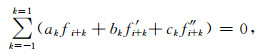(1)

1992年，Lele(1992)对Hermite公式进行了扩展，构造了求解一阶导数和二阶导数的紧致差分格式，表示为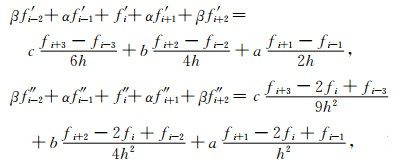(2)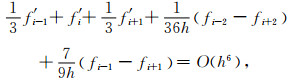(3)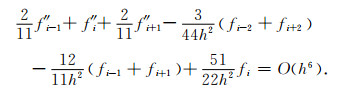(4)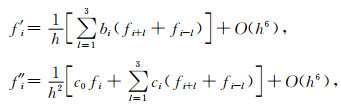(5)

Chu和Fan(1998)等构造了精度更高的三点六阶组合型紧致差分格式(Combined Compact Difference，以下简称CCD)：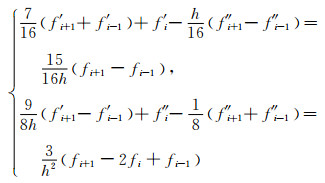(6)

2 声波方程组合型紧致有限差分方法分析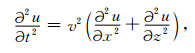(7)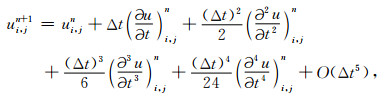(8)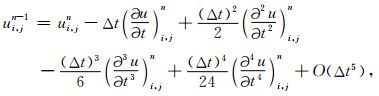(9)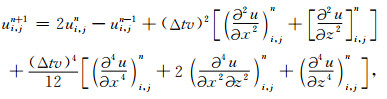(10)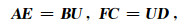(11)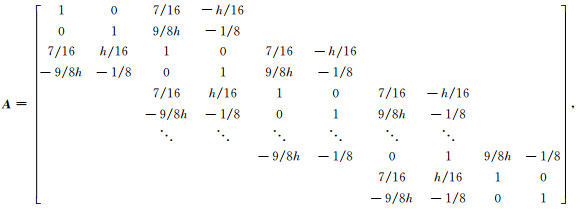(12)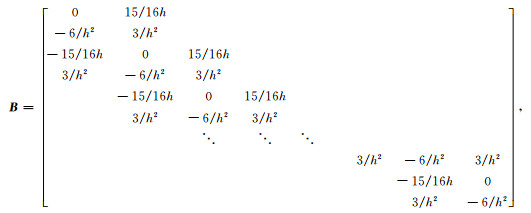(13)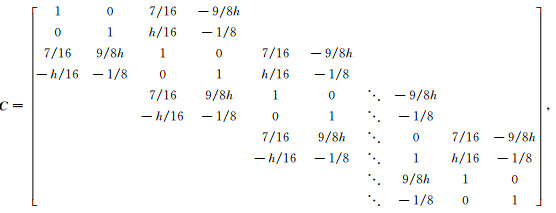(14)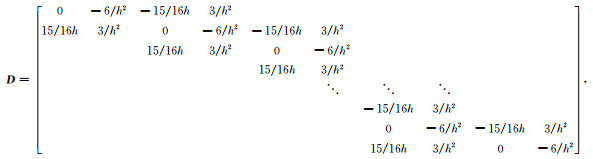(15)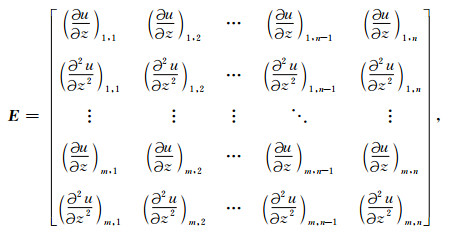(16)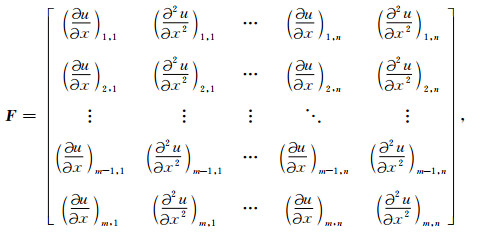(17)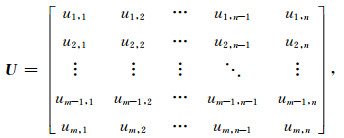(18)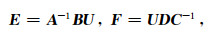(19)

2.1 精度分析表 1 一阶和二阶导数不同差分方法的截断误差比较 Table 1 Truncation errors in various difference schemes for the first and second derivative calculations

2.2 误差分析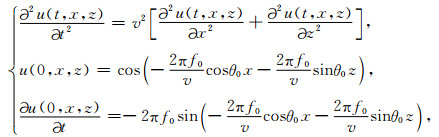(20)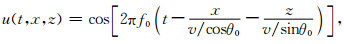(21)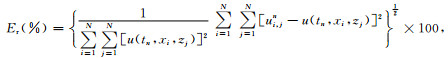(22)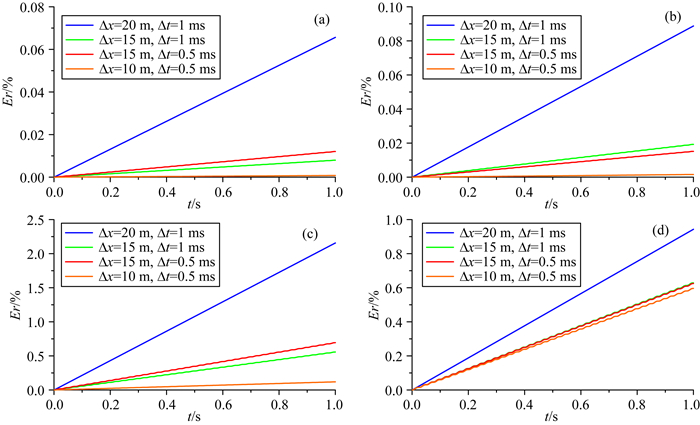图 1 四种数值模拟算法的相对误差曲线 (a) CCD; (b) CD; (c) ONAD; (d) CFD. Fig. 1 Relative error-time curves of the numerical simulation using four different methods and models (a) CCD; (b) CD; (c) ONAD; (d) CFD.表 2 四种方法在不同情况下的最大相对误差(%)比较 Table 2 Relative errors in four different schemes and parameters
2.3 频散分析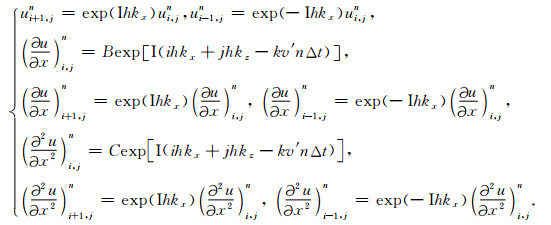(23)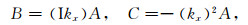(24)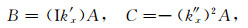(25)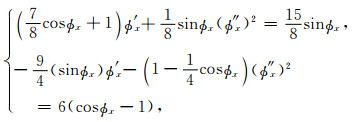(26)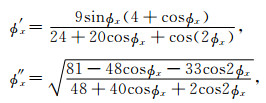(27)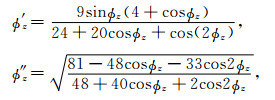(28)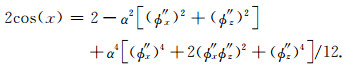(29)(30)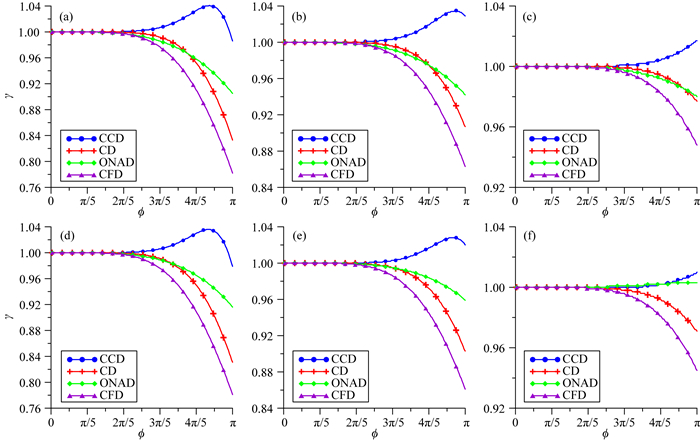图 2 不同差分方法的速度比曲线 (a) θ=0, α=0.25; (b) θ=π/8, α=0.25; (c) θ=π/4, α=0.25; (d) θ=0, α=0.45; (e) θ=π/8, α=0.45; (f) θ=π/4, α=0.45. Fig. 2 Velocity ratio curves of the numerical simulation in different methods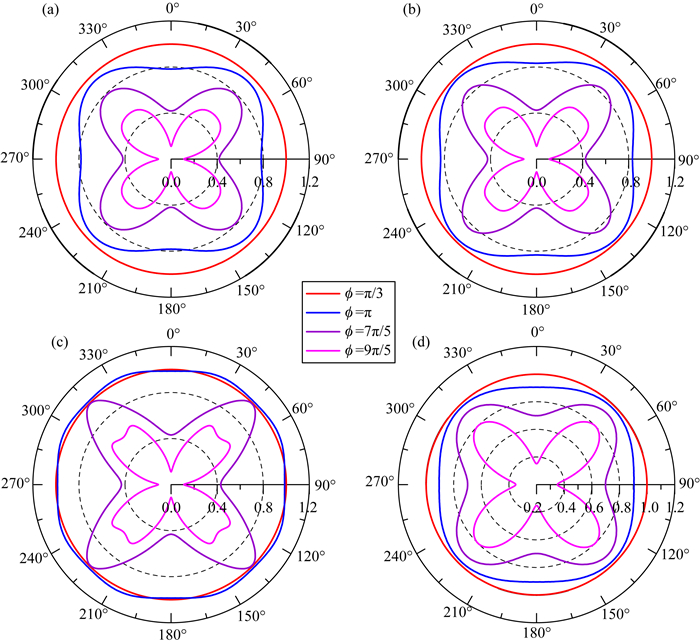图 3 不同差分方法的方位角-速度比曲线 (a) CFD; (b) CD; (c) CCD; (d) ONAD. Fig. 3 Azimuth-speed ratio curves of the numerical simulation in different methods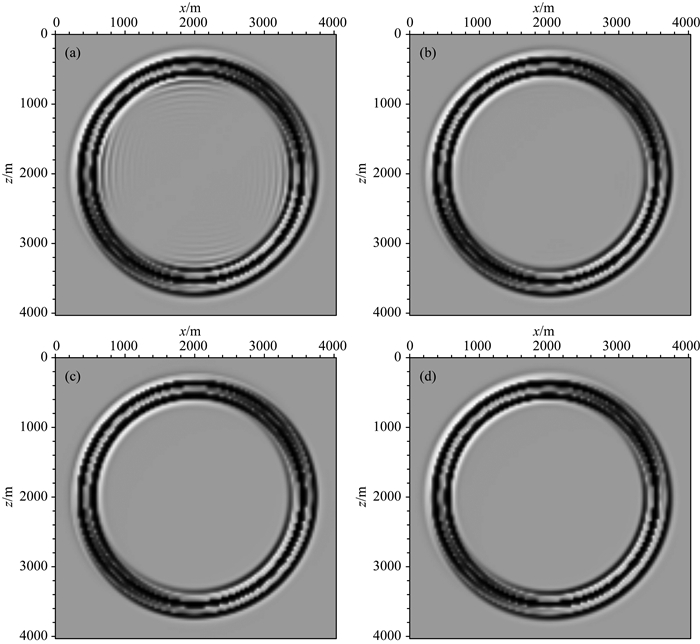图 4 空间网格32 m，时间步长1 ms，420 ms时刻波场快照 (a) CFD; (b) CD; (c) CCD; (d) ONAD. Fig. 4 Snapshots at 440ms: Δx=32 m, Δt=1 ms
2.4 稳定性分析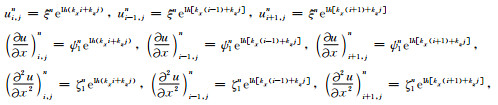(31)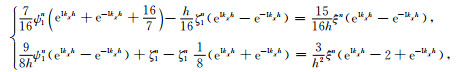(32)

θ1=kxh，-π/2≤θ1π/2，并利用欧拉公式，将上式化简可得：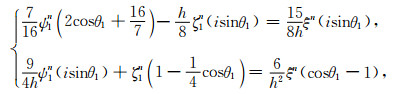(33)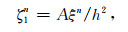(34)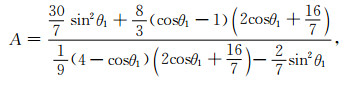(35)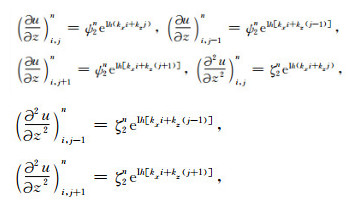(36)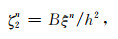(37)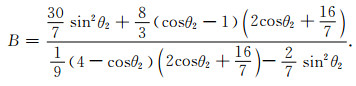(38)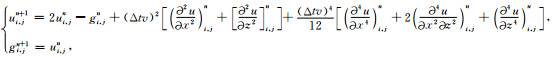(39)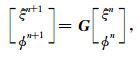(40)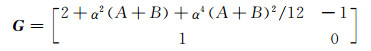(41)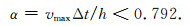(42)

2.5 计算效率分析表 3 不同方法计算效率比较 Table 3 Comparison of computational efficiency between different methods

3 弹性波方程组合型紧致有限差分方法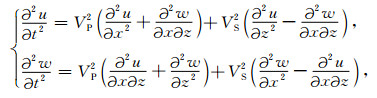(43)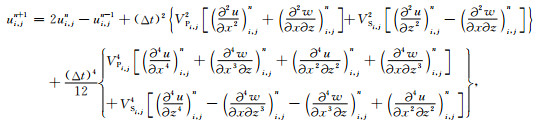(44)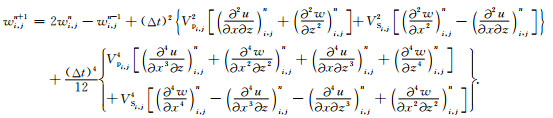(45)

4 模型试算

4.1 均匀介质模型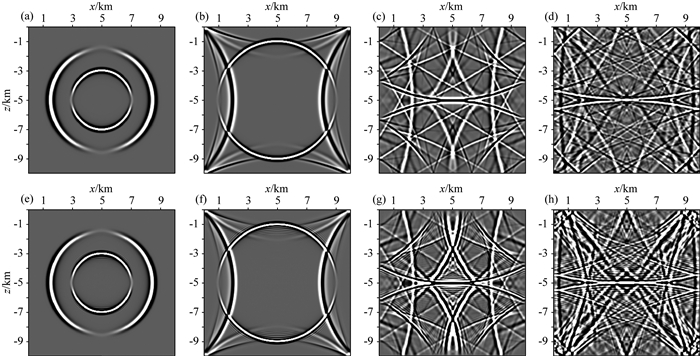图 5 均匀介质模型弹性波数值模拟波场快照 (a) CCD(1 s); (b) CCD(2 s); (c) CCD(5 s); (d) CCD(10 s); (e) CFD(1 s); (f) CFD(2 s); (g) CFD(5 s); (h) CFD(10 s). Fig. 5 Wave field snapshots using elastic wave equation in homogeneous medium (a—d) are the snapshots at 1, 2, 5, 10 s for the displacement component in X direction using CCD; (e—h) are the snapshots at 1, 2, 5, 10 s for the displacement component in X direction using CFD.
4.2 水平层状介质模型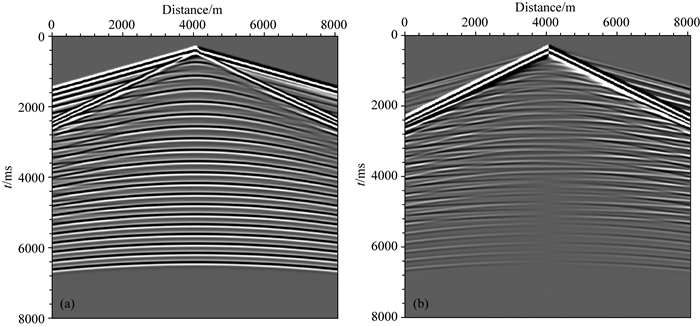图 6 水平层状介质模型弹性波方程数值模拟地面地震记录 (a) X方向位移分量；(b) Z方向位移分量. Fig. 6 Seismic records of the model′s surface using elastic wave equation in horizontal layers media (a) Displacement component in X direction; (b) Displacement component in Z direction.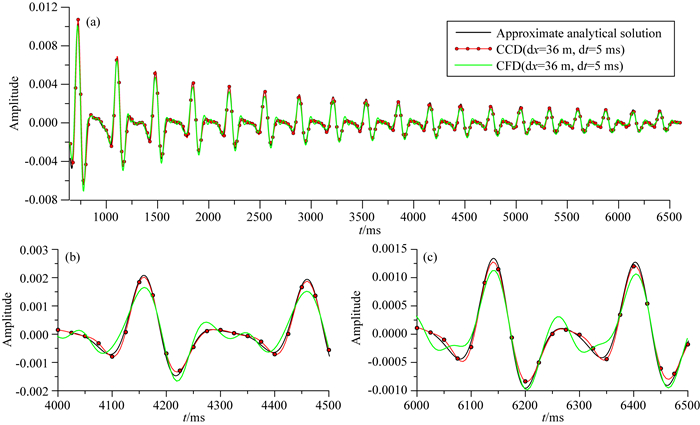图 7 接收点(4 km，0 km)处接收到的反射波水平位移波形图 (a)整道反射波记录对比；(b) 4000~4500 ms局部放大对比；(c) 6000~6500 ms局部放大对比.其中，红色加点实线是粗网格条件下的CCD数值结果，绿色实线是粗网格条件下的CFD数值结果，黑色实线是细网格条件下的近似理论解. Fig. 7 The horizontal displacement of reflected wave received at receiver (4 km, 0 km) (a) Comparison of the whole reflection wave record; (b) Comparison of the local amplification within 4000~4500 ms; (c) Comparison of the local amplification within 6000~6500 ms.The red dot solid line denotes the solution by CCD under coarse grid condition, and the green solid line denotes the solution by CFD under coarse grid condition, the black solid line denotes the approximate theoretical solution by CFD under tiny grid condition.

5 结论与建议

References
 Adams N A, Shariff K. 1996. A high-resolution hybrid compact-ENO scheme for Shock-Turbulence interaction problems. J. Comput. Phys., 127(1): 27-51. DOI:10.1006/jcph.1996.0156 Alterman Z, Karal F C Jr. 1968. Propagation of elastic waves in layered media by finite difference methods. Bull. Seismol. Soc. Am., 58(1): 367-398. Berenger J P. 1994. A perfectly matched layer for the absorption of electromagnetic waves. J. Comput. Phys., 114(2): 185-200. DOI:10.1006/jcph.1994.1159 Chen J B. 2006. Modeling the scalar wave equation with Nystr m methods. Geophysics, 71(5): T151-T158. DOI:10.1190/1.2335505 Cheng J B, Kang W, Wang T F. 2013. Description of qP-wave propagation in anisotropic media, Part Ⅰ:Pseudo-pure-mode wave equations. Chinese Journal of Geophysics (in Chinese), 56(10): 3474-3486. Chu C L, Phillips C, Stoffa P L. 2010. Frequency domain modeling using implicit spatial finite difference operators. SEG Technical Program Expanded Abstracts: 3076-3080. DOI:10.1190/SEGEAB.29 Chu P C, Fan C W. 1998. A three-point combined compact difference scheme. J. Comput. Phys., 140(2): 370-399. DOI:10.1006/jcph.1998.5899 Dennis S C R, Hudson J D. 1989. Compact h4 finite-difference approximations to operators of Navier-Stokes type. J. Comput. Phys., 85(2): 390-416. DOI:10.1016/0021-9991(89)90156-3 Dong L G, Ma Z T, Cao J Z, et al. 2000a. A staggered-grid high-order difference method of one-order elastic wave equation. Chinese Journal of Geophysics (in Chinese), 43(3): 411-419. DOI:10.3321/j.issn:0001-5733.2000.03.015 Dong L G, Ma Z T, Cao J Z. 2000b. A study on stability of the staggered-grid high-order difference method of first-order elastic wave equation. Chinese Journal of Geophysics (in Chinese), 43(6): 856-864. DOI:10.3321/j.issn:0001-5733.2000.06.015 Du Q Z, Li B, Hou B. 2009. Numerical modeling of seismic wavefields in transversely isotropic media with a compact staggered-grid finite difference scheme. Appl. Geophys., 6(1): 42-49. DOI:10.1007/s11770-009-0008-z Du Q Z, Guo C F, Gong X F. 2015. Hybrid PS/FD numerical simulation and stability analysis of pure P-wave propagation in VTI media. Chinese Journal of Geophysics (in Chinese), 58(4): 1290-1304. DOI:10.6038/cjg20150417 Fletcher R, Du X, Fowler P J. 2008. A new pseudo-acoustic wave equation for TI media.//Proceedings of 2008 SEG Annual Meeting. Las Vegas, Nevada: SEG, 2082-2086. http://www.mendeley.com/catalog/new-pseudoacoustic-wave-equation-ti-media/ Gao Y J, Zhang J H, Yao Z X. 2016. Third-order symplectic integration method with inverse time dispersion transform for long-term simulation. J. Comput. Phys., 314: 436-449. DOI:10.1016/j.jcp.2016.03.031 Graves R W. 1996. Simulating seismic wave propagation in 3D elastic media using staggered-grid finite differences. Bull. Seismol. Soc. Am., 86(4): 1091-1106. He X J, Yang D H, Wu H. 2015. A weighted Runge-Kutta discontinuous Galerkin method for wavefield modelling. Geophys. J. Int., 200(3): 1389-1410. DOI:10.1093/gji/ggu487 Huang C, Dong L G. 2009. Staggered-grid high-order finite-difference method in elastic wave simulation with variable grids and local time-steps. Chinese Journal of Geophysics (in Chinese), 52(11): 2870-2878. Jing H, Chen Y S, Yang D H, et al. 2017. Dispersion-relation preserving stereo-modeling method beyond Nyquist frequency for acoustic wave equation. Geophysics, 82(1): T1-T15. DOI:10.1190/geo2016-0104.1 Kosloff D, Pestana R C, Tal-Ezer H. 2010. Acoustic and elastic numerical wave simulations by recursive spatial derivative operators. Geophysics, 75(6): T167-T174. DOI:10.1190/1.3485217 Lele S K. 1992. Compact finite difference schemes with spectral-like resolution. J. Comput. Phys., 103(1): 16-42. DOI:10.1016/0021-9991(92)90324-R Li X F, Wang W S, Lu M W, et al. 2012. Structure-preserving modelling of elastic waves:A symplectic discrete singular convolution differentiator method. Geophys. J. Int, 188(3): 1382-1392. DOI:10.1111/gji.2012.188.issue-3 Li Z C, Yang F S, Wang X D. 2016. High-precision numerical simulation of first-order qP-waves in the transversely isotropic (TI) medium optimized by the LS-RSGFD method. Chinese Journal of Geophysics (in Chinese), 59(4): 1477-1490. DOI:10.6038/cjg20160428 Liu S L, Yang D H, Lan C, et al. 2017. Modified symplectic schemes with nearly-analytic discrete operators for acoustic wave simulations. Comput. Phys. Commun., 213: 52-63. DOI:10.1016/j.cpc.2016.12.002 Liu Y, Sen M K. 2009a. A practical implicit finite-difference method:Examples from seismic modelling. J. Geophys. Eng., 6(3): 231-249. DOI:10.1088/1742-2132/6/3/003 Liu Y, Sen M K. 2009b. An implicit staggered-grid finite-difference method for seismic modelling. Geophys. J. Int., 179(1): 459-474. DOI:10.1111/gji.2009.179.issue-1 Liu Y. 2013. Globally optimal finite-difference schemes based on least squares. Geophysics, 78(4): T113-T132. DOI:10.1190/geo2012-0480.1 Liu Y. 2014. An optimal 5-point scheme for frequency-domain scalar wave equation. J. Appl. Geophys., 108: 19-24. DOI:10.1016/j.jappgeo.2014.06.006 Liu Y S, Liu S L, Zhang M G, et al. 2012. An improved perfectly matched layer absorbing boundary condition for second order elastic wave equation. Progress in Geophysics (in Chinese), 27(5): 2113-2122. Ma X, Yang D H. 2017. A phase-preserving and low-dispersive symplectic partitioned Runge-Kutta method for solving seismic wave equations. Geophys. J. Int., 209(3): 1534-1557. DOI:10.1093/gji/ggx097 Pan G D, Abubakar A, Habashy M T. 2012. An effective perfectly matched layer design for acoustic fourth-order frequency-domain finite-difference scheme. Geophys. J. Int., 188(1): 211-222. DOI:10.1111/gji.2012.188.issue-1 Saenger E H, Bohlen T. 2004. Finite-difference modeling of viscoelastic and anisotropic wave propagation using the rotated staggered grid. Geophysics, 69(2): 583-591. DOI:10.1190/1.1707078 Saenger E H, Gold N, Shapiro S A. 2000. Modeling the propagation of elastic waves using a modified finite-difference grid. Wave Motion, 31(1): 77-92. DOI:10.1016/S0165-2125(99)00023-2 Song G J. 2008. The improved nearly analytic discrete method for 3D elastic equations and its numerical simulation [Master's thesis] (in Chinese). Beijing: Tsinghua University. Tang J, Wang F, Liu F L. 2016. 3D wave equation forward modeling based on three-level parallel acceleration. Oil Geophysical Prospecting (in Chinese), 51(5): 1049-1054. Tong P, Yang D H, Hua B L, et al. 2013. A high-order stereo-modeling method for solving wave equations. Bull. Seismol. Soc. Am., 103(2A): 811-833. DOI:10.1785/0120120144 Virieux J. 1986. P-SVwave propagation in heterogeneous media:Velocity-stress finite-difference method. Geophysics, 51(4): 889-901. DOI:10.1190/1.1442147 Wang L C, Chang X, Wang Y B. 2016. Forward modeling of pseudo P waves in TTI medium using staggered grid. Chinese Journal of Geophysics (in Chinese), 59(3): 1046-1058. DOI:10.6038/cjg20160325 Wang S Q, Yang D H, Yang K D. 2002. Compact finite difference scheme for elastic equations. Journal of Tsinghua University (Science and Technology) (in Chinese), 42(8): 1128-1131. Yang D H, Wang S Q, Zhang Z J, et al. 2003. n-times absorbing boundary conditions for compact finite-difference modeling of acoustic and elastic wave propagation in the 2D TI Medium. Bull. Seismol. Soc. Am., 93(6): 2389-2401. DOI:10.1785/0120020224 Yang D H, Wang N, Chen S, et al. 2009. An explicit method based on the implicit Runge-Kutta algorithm for solving wave equations. Bull. Seismol. Soc. Am., 99(6): 3340-3354. DOI:10.1785/0120080346 Yang D H, Wang L. 2010. A split-step algorithm for effectively suppressing the numerical dispersion for 3D seismic propagation modeling. Bull. Seismol. Soc. Am., 100(4): 1470-1484. DOI:10.1785/0120090200 Yang D H, Tong P, Deng X Y. 2012a. A central difference method with low numerical dispersion for solving the scalar wave equation. Geophysical Prospecting, 60(5): 885-905. DOI:10.1111/gpr.2012.60.issue-5 Yang D H, Wang N, Liu E. 2012b. A strong stability-preserving predictor-corrector method for the simulation of elastic wave propagation in anisotropic media. Communications in Computational Physics, 12(4): 1006-1032. DOI:10.4208/cicp.010111.230911a Yang D H, Wang M X, Ma X. 2014. Symplectic stereomodelling method for solving elastic wave equations in porous media. Geophys. J. Int., 196(1): 560-579. DOI:10.1093/gji/ggt393 Yang K D, Song G J, Li J S. 2011. FCT compact difference simulation of wave propagation based on the Biot and the squirt-flow coupling interaction. Chinese Journal of Geophysics (in Chinese), 54(5): 1348-1357. DOI:10.3969/j.issn.0001-5733.2011.05.024 Zhang J H, Yao Z X. 2013a. Optimized finite-difference operator for broadband seismic wave modeling. Geophysics, 78(1): A13-A18. DOI:10.1190/geo2012-0277.1 Zhang J H, Yao Z X. 2013b. Optimized explicit finite-difference schemes for spatial derivatives using maximum norm. J. Comput. Phys., 250: 511-526. DOI:10.1016/j.jcp.2013.04.029 Zhang W S. 2006. Finite Difference Methods for Partial Differential Equations in Science Computation. Beijing: Higher Education Press. Zhou B, Greenhalgh S A. 2003. Crosshole seismic inversion with normalized full-waveform amplitude data. Geophysics, 68(4): 1320-1330. DOI:10.1190/1.1598125 Zhou Y J, Yang D H, Ma X, et al. 2015. An effective method to suppress numerical dispersion in 2D acoustic and elastic modelling using a high-order Padé approximation. J. Geophys. Eng., 12(1): 114-129. DOI:10.1088/1742-2132/12/1/114 程玖兵, 康玮, 王腾飞. 2013. 各向异性介质qP波传播描述Ⅰ:伪纯模式波动方程. 地球物理学报, 56(10): 3474-3486. DOI:10.6038/cjg20131022 董良国, 马在田, 曹景忠, 等. 2000a. 一阶弹性波方程交错网格高阶差分解法. 地球物理学报, 43(3): 411-419. DOI:10.3321/j.issn:0001-5733.2000.03.015 董良国, 马在田, 曹景忠. 2000b. 一阶弹性波方程交错网格高阶差分解法稳定性研究. 地球物理学报, 43(6): 856-864. DOI:10.3321/j.issn:0001-5733.2000.06.015 杜启振, 郭成锋, 公绪飞. 2015. VTI介质纯P波混合法正演模拟及稳定性分析. 地球物理学报, 58(4): 1290-1304. DOI:10.6038/cjg20150417 黄超, 董良国. 2009. 可变网格与局部时间步长的交错网格高阶差分弹性波模拟. 地球物理学报, 52(11): 2870-2878. 李振春, 杨富森, 王小丹. 2016. 基于LS-RSGFD方法优化的横向各向同性(TI)介质一阶qP波高精度数值模拟. 地球物理学报, 59(4): 1477-1490. DOI:10.6038/cjg20160428 刘有山, 刘少林, 张美根, 等. 2012. 一种改进的二阶弹性波动方程的最佳匹配层吸收边界条件. 地球物理学进展, 27(5): 2113-2122. 宋国杰. 2008.三维弹性波方程的改进近似解析离散化方法及波场模拟[硕士论文].北京: 清华大学. 唐佳, 王凡, 刘福烈. 2016. 三维波动方程正演的三级并行加速. 石油地球物理勘探, 51(5): 1049-1054. 王璐琛, 常旭, 王一博. 2016. TTI介质的交错网格伪P波正演方法. 地球物理学报, 59(3): 1046-1058. DOI:10.6038/cjg20160325 王书强, 杨顶辉, 杨宽德. 2002. 弹性波方程的紧致差分方法. 清华大学学报(自然科学版), 42(8): 1128-1131. DOI:10.3321/j.issn:1000-0054.2002.08.037 杨宽德, 宋国杰, 李静爽. 2011. Biot流动和喷射流动耦合作用下波传播的FCT紧致差分模拟. 地球物理学报, 54(5): 1348-1357. DOI:10.3969/j.issn.0001-5733.2011.05.024 张文生. 2006. 科学计算中的偏微分方程有限差分法. 北京: 高等教育出版社.